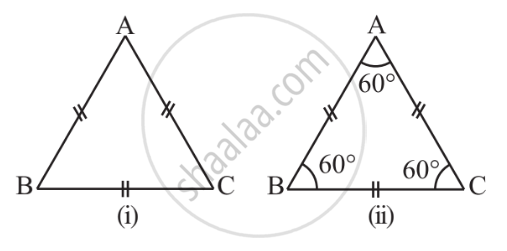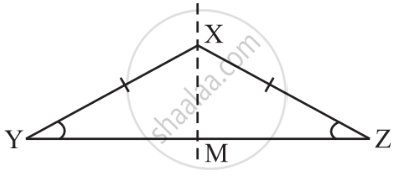# Some Special Types of Triangles - Equilateral and Isosceles Triangles

## Definition

Equilateral Triangle: A triangle in which all three sides are of equal lengths is called an equilateral triangle.

Isosceles Triangle: A triangle in which two sides are of equal lengths is called an isosceles triangle.

# Equilateral Triangle:

• A triangle in which all three sides are of equal lengths is called an equilateral triangle.

• A triangle is said to be equilateral if each one of its sides has the same length.

• In an equilateral triangle, each angle has measure 60°.# Isosceles Triangle:

• A triangle in which two sides are of equal lengths is called an isosceles triangle.

• A triangle is said to be isosceles if at least any two of its sides are of the same length.

• The non-equal side of an isosceles triangle is called its base.

• The base angles of an isosceles triangle have equal measure.

• An equilateral triangle satisfies all the properties of an isosceles triangle, whereas it is not necessary for an isosceles triangle to satisfy all the properties of an equilateral triangle.If you would like to contribute notes or other learning material, please submit them using the button below.

### Shaalaa.com

What is an Equilateral Triangle & an Isosceles Triangle? [00:18:20]
S
0%